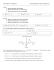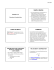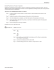C3 Worksheet C - A Level Maths Help

Transcription

C3 Worksheet C - A Level Maths Help
C3
1
Worksheet C
NUMERICAL METHODS
a Show that the equation x3 − 7x − 11 = 0 has a real root in the interval (3, 4).
b Using the iterative formula xn + 1 =
7 + 11
x , with x0 = 3.2, find x1, x2 and x3, giving the value
n
of x3 correct to 2 decimal places.
2
f(x) ≡ 4 cosec x − 5 + 2x.
a Find the values of f(4) and f(5).
b Hence show that the equation f(x) = 0 has a root in the interval (4, 5).
The iterative formula xn + 1 = a +
b
sin xn
, where a and b are constants, is used to find this root.
c Find the values of a and b.
d Starting with x0 = 4.5, use the iterative formula with your values of a and b to find 3 further
approximations of the root, giving your final answer correct to 3 decimal places.
3
y
y = ex + x2 − 4
O
B
A
x
The diagram shows the curve y = ex + x2 − 4. The curve intersects the y-axis at the point A and
has a stationary point at B.
dy
a Find
.
dx
b Find an equation for the tangent to the curve at A.
c Show that the x-coordinate of B lies in the interval [−0.4, −0.3].
d Using an iteration process based on the rearrangement x =
x-coordinate of B correct to 3 decimal places.
4
1
3
(x − ex), with x0 = −0.3, find the
a On the same set of axes, sketch the curves y = cos x and y = x2 for − π2 < x <
π
2
.
b Show that the equation cos x − x2 = 0 has exactly one positive and one negative real root.
c Show that the positive real root lies in the interval [0.8, 0.9].
d Use the iteration formula xn + 1 =
root correct to 2 decimal places.
5
cos xn and the starting value x0 = 0.8 to find the positive
f(x) ≡ e5 − 2x − x5.
Show that the equation f(x) = 0
a has a root in the interval (1.4, 1.5),
b can be written as x = e1 − kx, stating the value of k.
c Using the iteration formula xn + 1 = e1 − kxn , with x0 = 1.5 and the value of k found in part b,
find x1, x2 and x3. Give the value of x3 correct to 3 decimal places.
 Solomon Press
C3
NUMERICAL METHODS
Worksheet C continued
f : x → 2x + x3 − 5, x ∈ .
6
a Show that there is a solution of the equation f(x) = 0 in the interval 1.3 < x < 1.4
3
b Using the iterative formula xn + 1 =
5 − 2 xn , with x0 = 1.4, find x1, x2, x3 and x4.
c Hence write down an approximation for this solution of the equation f(x) = 0 to an
appropriate degree of accuracy.
Another attempt is made to find the solution using the iterative formula xn + 1 =
3
ln (5 − xn )
ln 2
.
d Describe the outcome of this attempt.
f(x) = 2x3 + 4x − 9.
7
a Find f ′(x).
b Hence show that the equation f(x) = 0 has exactly one real root.
c Show that this root lies in the interval (1.2, 1.3).
d Use the iterative formula xn + 1 =
correct to 2 decimal places.
3
4.5 − 2 xn , with x0 = 1.2, to find the root of f(x) = 0
8
y
y=x
B
y = 3x + ln x − x2
O A
x
The diagram shows part of the curve with equation y = 3x + ln x − x2 and the line y = x.
Given that the curve and line intersect at the points A and B, show that
a the x-coordinates of A and B are the solutions of the equation x = e x
2
−2 x
,
b the x-coordinate of A lies in the interval (0.4, 0.5),
c the x-coordinate of B lies in the interval (2.3, 2.4).
d Use the iteration formula xn + 1 = e xn
to 2 decimal places.
2
− 2 xn
, with x0 = 0.5, to find the x-coordinate of A correct
9
a On the same set of axes, sketch the graphs of y = x4 and y = 5x + 2.
b Show that the equation x4 − 5x − 2 = 0 has exactly one positive and one negative real root.
c Use the iteration formula xn + 1 = 4 5 xn + 2 , with x0 = 1.8, to find x1, x2, x3 and x4, giving the
value of x4 correct to 3 decimal places.
d Show that the equation x4 − 5x − 2 = 0 can be written in the form x =
values of a and b.
e Use the iteration formula xn + 1 =
a
3
xn + b
a
x +b
3
, stating the
, with x0 = −0.4 and your values of a and b, to find
the negative real root of the equation correct to 4 decimal places.
 Solomon Press

3 sample exam paper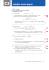Math Wuzzles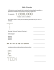Dewey Decimal Classification System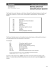MATH 148 FINAL EXAM SAMPLE PROBLEMS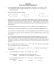Math 121 - Sample Final Exam - F07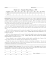Test 3 Review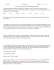Small-Sample C.I.s for one- sample, two-sample and (matched) paired data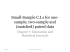WISCONSIN MIDDLE SCHOOL STATE MATHEMATICS MEET WISCONSIN MATHEMATICS COUNCIL Sample Questions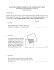11.4 So what is this thing called pi? student activity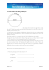AP Calculus AB – Worksheet 83 The Second Derivative and The